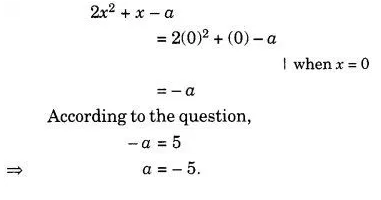# What should be the value of a if the value of 2x2 + x – a equals to 5, when x = 0?

What should be the value of a if the value of 2x2 + x – a equals to 5, when x = 0 ?